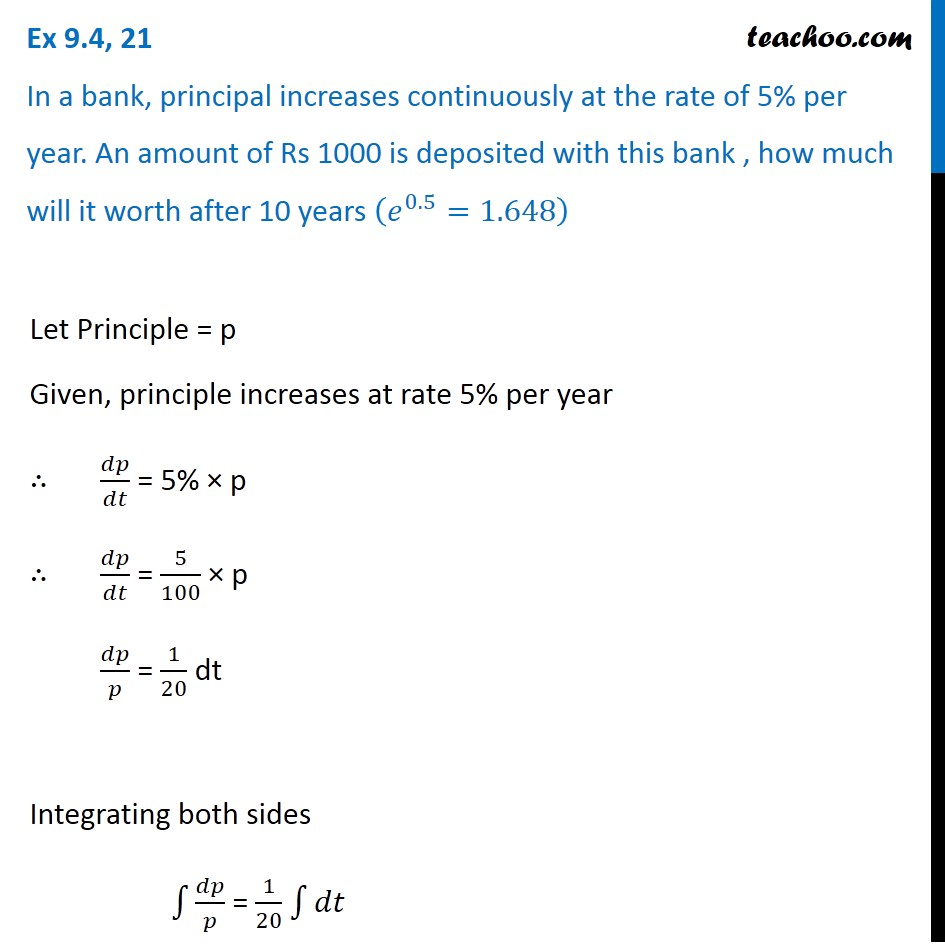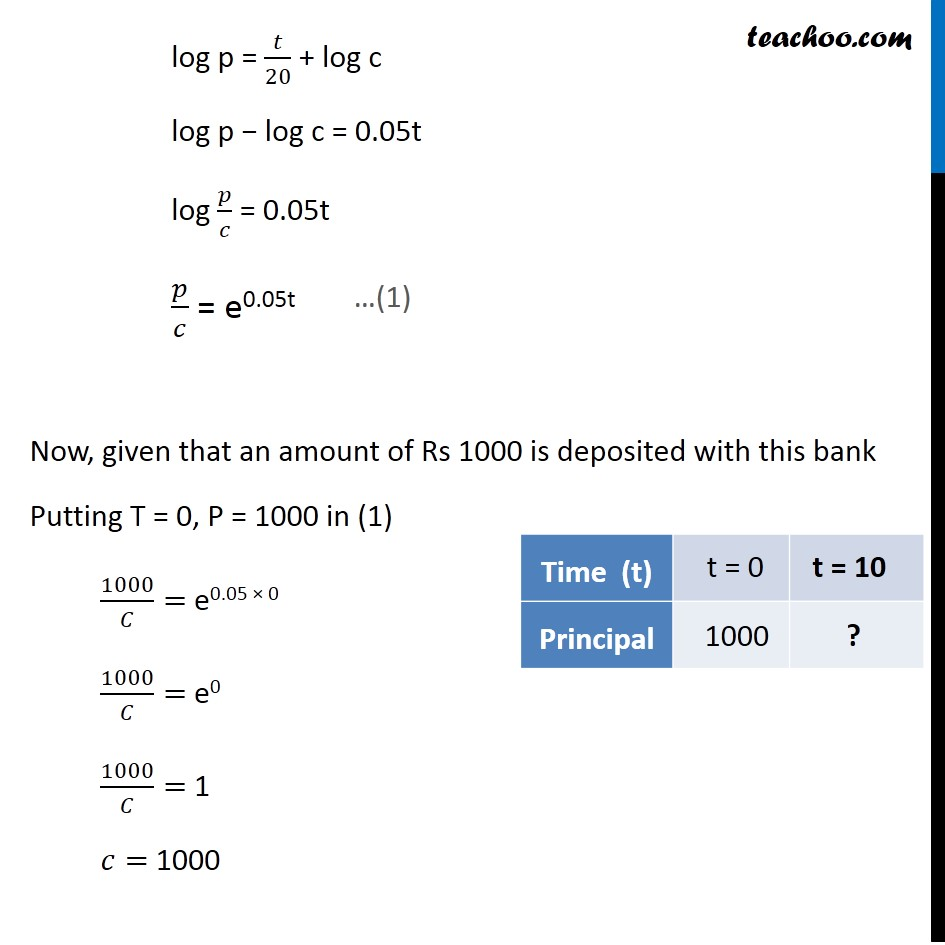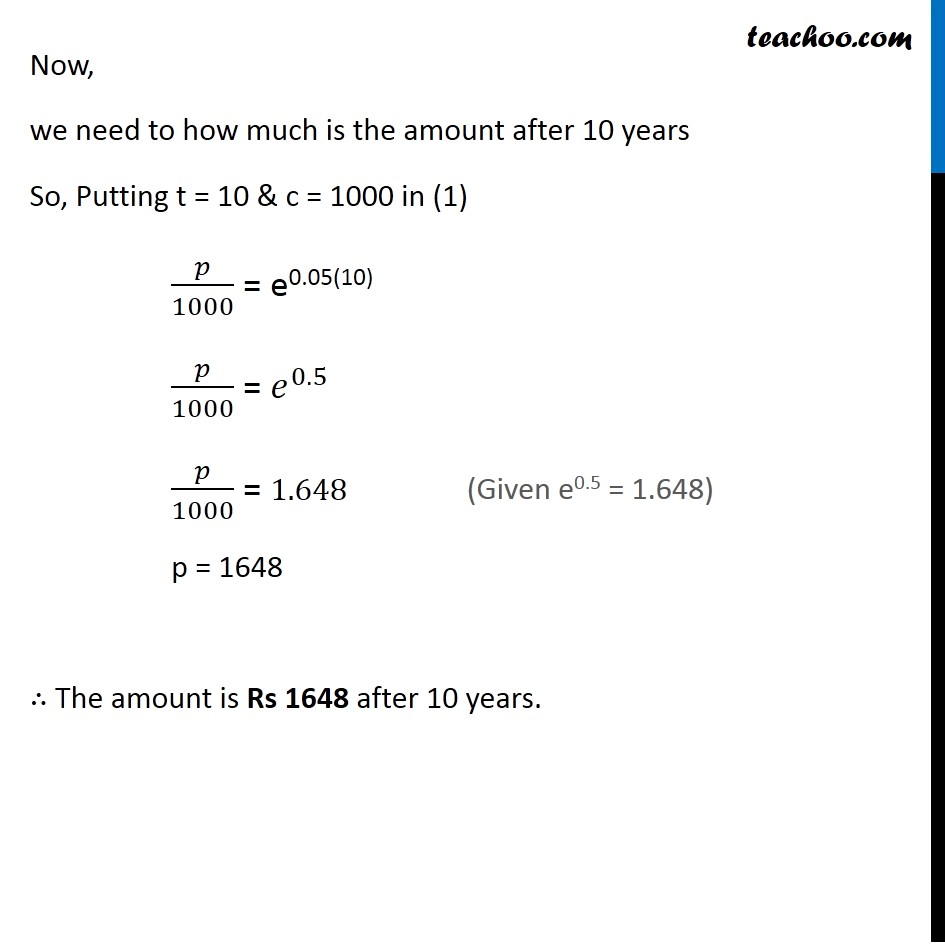Ex 9.3

Chapter 9 Class 12 Differential Equations
Serial order wiseLearn in your speed, with individual attention - Teachoo Maths 1-on-1 Class

### Transcript

Ex 9.3, 21 In a bank, principal increases continuously at the rate of 5% per year. An amount of Rs 1000 is deposited with this bank , how much will it worth after 10 years (𝑒^0.5=1.648) Let Principle = p Given, principle increases at rate 5% per year ∴ 𝑑𝑝/𝑑𝑡 = 5% × p ∴ 𝑑𝑝/𝑑𝑡 = 5/100 × p 𝑑𝑝/𝑝 = 1/20 dt Integrating both sides ∫1▒𝑑𝑝/𝑝 = 1/20 ∫1▒𝑑𝑡 log p = 𝑡/20 + log c log p − log c = 0.05t log 𝑝/𝑐 = 0.05t 𝑝/𝑐 = e0.05t Now, given that an amount of Rs 1000 is deposited with this bank Putting T = 0, P = 1000 in (1) 1000/𝐶= e0.05 × 0 1000/𝐶= e0 1000/𝐶= 1 𝑐= 1000 …(1) Now, we need to how much is the amount after 10 years So, Putting t = 10 & c = 1000 in (1) 𝑝/1000 = e0.05(10) 𝑝/1000 = 𝑒^0.5 𝑝/1000 = 1.648 p = 1648 ∴ The amount is Rs 1648 after 10 years. (Given e0.5 = 1.648)